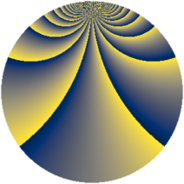# Properties

 Label 1792.2.uLevel $1792$ Weight $2$ Character orbit 1792.u Rep. character $\chi_{1792}(225,\cdot)$ Character field $\Q(\zeta_{8})$ Dimension $192$ Sturm bound $512$

# Learn more

## Defining parameters

 Level: $$N$$ $$=$$ $$1792 = 2^{8} \cdot 7$$ Weight: $$k$$ $$=$$ $$2$$ Character orbit: $$[\chi]$$ $$=$$ 1792.u (of order $$8$$ and degree $$4$$) Character conductor: $$\operatorname{cond}(\chi)$$ $$=$$ $$32$$ Character field: $$\Q(\zeta_{8})$$ Sturm bound: $$512$$

## Dimensions

The following table gives the dimensions of various subspaces of $$M_{2}(1792, [\chi])$$.

Total New Old
Modular forms 1088 192 896
Cusp forms 960 192 768
Eisenstein series 128 0 128

## Trace form

 $$192q + O(q^{10})$$ $$192q + 32q^{53} + 128q^{61} + 128q^{69} + 32q^{77} + O(q^{100})$$

## Decomposition of $$S_{2}^{\mathrm{new}}(1792, [\chi])$$ into newform subspaces

The newforms in this space have not yet been added to the LMFDB.

## Decomposition of $$S_{2}^{\mathrm{old}}(1792, [\chi])$$ into lower level spaces

$$S_{2}^{\mathrm{old}}(1792, [\chi]) \cong$$ $$S_{2}^{\mathrm{new}}(32, [\chi])$$$$^{\oplus 8}$$$$\oplus$$$$S_{2}^{\mathrm{new}}(128, [\chi])$$$$^{\oplus 4}$$$$\oplus$$$$S_{2}^{\mathrm{new}}(224, [\chi])$$$$^{\oplus 4}$$$$\oplus$$$$S_{2}^{\mathrm{new}}(256, [\chi])$$$$^{\oplus 2}$$$$\oplus$$$$S_{2}^{\mathrm{new}}(896, [\chi])$$$$^{\oplus 2}$$